How Cheenta works to ensure student success?
Explore the Back-StoryHere are the questions asked in Regional Math Olympiad 2017 and their solutions. Try to solve it first and then see the solutions.

RMO 2017, Problem 1:

Let AOB be a given angle less thanand let P be an interior point of the angular region determined by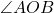. Show, with proof, how to construct, using only ruler and compass, a line segment CD passing through P such that C lies on the ray OA and D lies on the ray OB and CP:PD = 1:2.

RMO 2017, Problem 2:

Show that the equation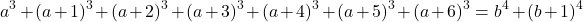has no solutions in integer a, b.

RMO 2017, Problem 3:

Let P(x)=x2+12x+b$P(x) = x^2 + \frac {1}{2} x + b$ and Q(x)=x2+cx+d$Q(x) = x^2 + cx + d$ be two polynomials with real coefficients such that P(x) Q(x) = Q(P(x)) for all real x. Find all real roots of P(Q(x)) = 0

RMO 2017, Problem 4:

Consider n2$n^2$ unit squares in the xy-plane centered at the point (i, j) with integer coordinates, 1in$1 \le i \le n$ , 1jn$1 \le j \le n$ . It is required to color each unit square in such a way that whenever 1≤ i < j and 1 ≤ k < l ≤ n the three squares with centers at (i, k), (j, k) , (j, l) have distinct colours. What is the least possible colours needed?

RMO 2017, Problem 5:

Let Ω$\Omega$ be a circle with a chord AB which is not a diameter. Let Γ1$\Gamma_1$ be a circle on one side of AB such that it is tangent to AB at C and internally tangent to Ω$\Omega$ at D. Likewise let Γ2$\Gamma_2$ be a circle on the other side of AB such that it is tangent to AB at E and internally tangent to Ω$\Omega$ at F. Suppose the line DC intersects Ω$\Omega$ at XD$X \neq D$ and the line FE intersects Ω$\Omega$ at YF$Y \neq F$. Prove that XY is a diameter of Ω

RMO 2017, Problem 6:

Let x, y, z be real numbers, each greater than 1. Prove that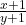++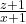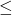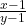+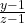+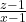.

~ Discussion by Souvik Mondal & Writabrata Bhattacharya (Associate Faculty - Cheenta)

Here are the questions asked in Regional Math Olympiad 2017 and their solutions. Try to solve it first and then see the solutions.

RMO 2017, Problem 1:

Let AOB be a given angle less thanand let P be an interior point of the angular region determined by. Show, with proof, how to construct, using only ruler and compass, a line segment CD passing through P such that C lies on the ray OA and D lies on the ray OB and CP:PD = 1:2.

RMO 2017, Problem 2:

Show that the equationhas no solutions in integer a, b.

RMO 2017, Problem 3:

Let P(x)=x2+12x+b$P(x) = x^2 + \frac {1}{2} x + b$ and Q(x)=x2+cx+d$Q(x) = x^2 + cx + d$ be two polynomials with real coefficients such that P(x) Q(x) = Q(P(x)) for all real x. Find all real roots of P(Q(x)) = 0

RMO 2017, Problem 4:

Consider n2$n^2$ unit squares in the xy-plane centered at the point (i, j) with integer coordinates, 1in$1 \le i \le n$ , 1jn$1 \le j \le n$ . It is required to color each unit square in such a way that whenever 1≤ i < j and 1 ≤ k < l ≤ n the three squares with centers at (i, k), (j, k) , (j, l) have distinct colours. What is the least possible colours needed?

RMO 2017, Problem 5:

Let Ω$\Omega$ be a circle with a chord AB which is not a diameter. Let Γ1$\Gamma_1$ be a circle on one side of AB such that it is tangent to AB at C and internally tangent to Ω$\Omega$ at D. Likewise let Γ2$\Gamma_2$ be a circle on the other side of AB such that it is tangent to AB at E and internally tangent to Ω$\Omega$ at F. Suppose the line DC intersects Ω$\Omega$ at XD$X \neq D$ and the line FE intersects Ω$\Omega$ at YF$Y \neq F$. Prove that XY is a diameter of Ω

RMO 2017, Problem 6:

Let x, y, z be real numbers, each greater than 1. Prove that++++.

~ Discussion by Souvik Mondal & Writabrata Bhattacharya (Associate Faculty - Cheenta)

This site uses Akismet to reduce spam. Learn how your comment data is processed.

### Knowledge Partner